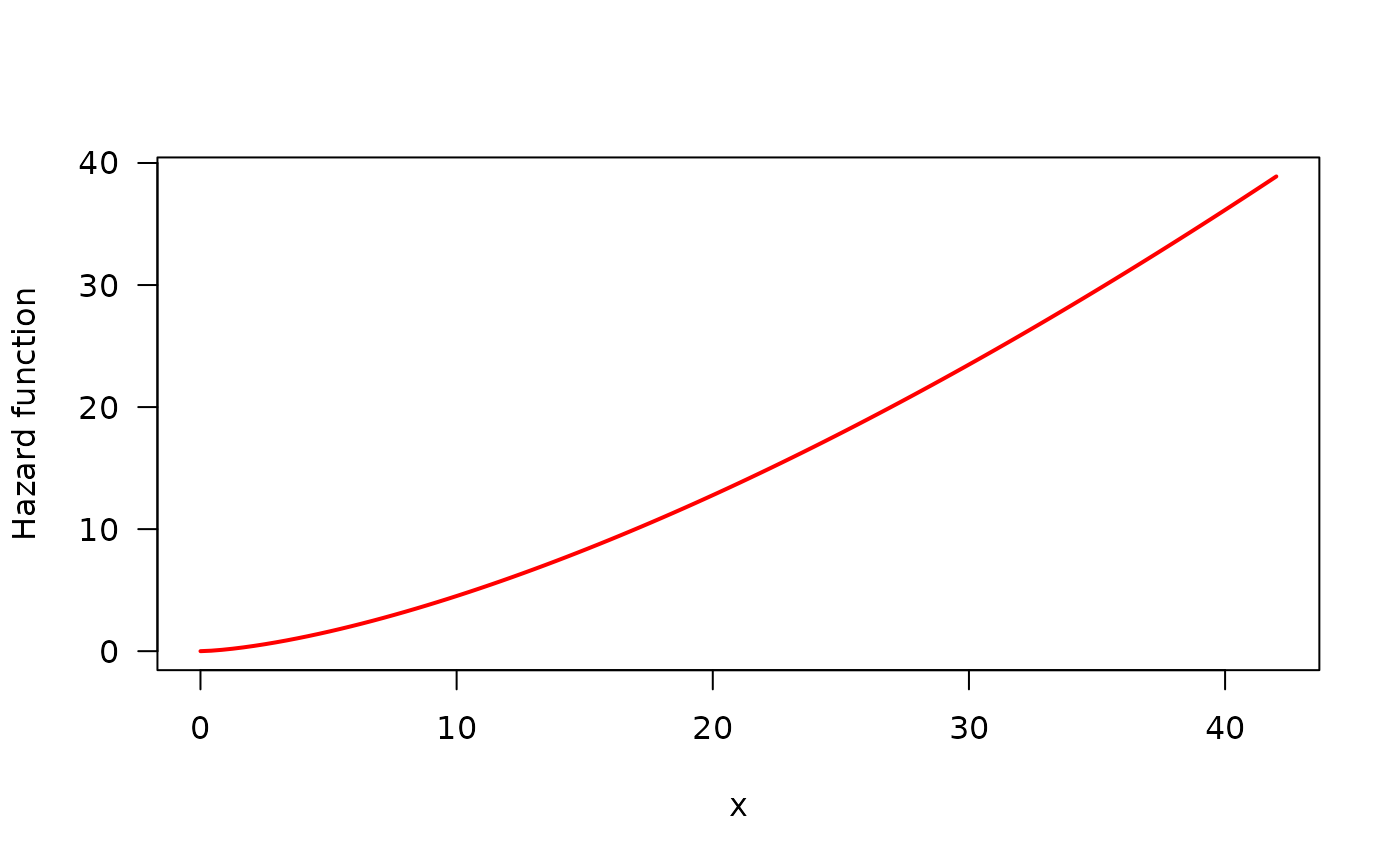Displays the estimated hazard shape given a HazardShape object.

## Usage

# S3 method for HazardShape
print(x, ...)

## Arguments

x

an object of class HazardShape, generated with TTT_hazard_shape.

...

further arguments passed to or from other methods.

## Author

Jaime Mosquera Gutiérrez jmosquerag@unal.edu.co

## Examples

#--------------------------------------------------------------------------------
# Example 1: Increasing hazard and its corresponding TTT plot with simulated data

hweibull <- function(x, shape, scale){
dweibull(x, shape, scale)/pweibull(x, shape, scale, lower.tail = FALSE)
}
curve(hweibull(x, shape = 2.5, scale = pi), from = 0, to = 42,
col = "red", ylab = "Hazard function", las = 1, lwd = 2)y <- rweibull(n = 50, shape = 2.5, scale = pi)
my_initial_guess <- TTT_hazard_shape(formula = y ~ 1)
print(my_initial_guess)
#> --------------------------------------------------------------------
#> Hazard shape: Increasing
#> --------------------------------------------------------------------
#> Warning: Non-parametric estimate for Empirical TTT is irregular.
#> Please, use the 'plot()' method to see the TTT shape

#--------------------------------------------------------------------------------